20.2
20.1
19.2
19.1
18.2
18.1
17.2

# Axis Scale Types

This topic explains how to specify axis scale options for different types of series point arguments and values (Numerical, Date-Time, Time-Span, and Qualitative). Scale options allow you to set scale parameters as an axis measurement unit, grid spacing, grid offset, an aggregate function, and so on.

The topic consists of the following sections:

##### TIP
1. Refer to the Choosing between DateTime, DateTimeOffset, TimeSpan, and TimeZoneInfo document (see the The DateTime structure and The TimeSpan structure sections) for more information about DateTime and TimeSpan values.

2. To format text labels the Chart displays on an axis, use the AxisLabel.TextPattern property.

3. You can configure an axis range to limit the axis scale value range.

## Determine X-Axis Scale Type Automatically

The Chart Control determines an x-axis's scale type based on underlying data (the SeriesBase.ArgumentScaleType property is set to Auto). Note that this operation requires additional CPU and RAM resources. To explicitly specify the argument type, use the SeriesBase.ArgumentScaleType property. See the following help topic for more information: Performance: Automatic Calculations.

## Numerical, Date-Time and Time-Span Axis Scale Modes

The scale mode defines how the Chart aggregates series point values. The following modes are available for x-axes. Y-axes always have a continuous scale.

Scale Mode

Description

Automatic

Automatic aggregation is applied to data based on the data set's values, the Chart Control's current size, and the zoom level.

Continuous

The Chart Control does not aggregate data.

Manual

An axis scale is divided into intervals based on the measurement unit (NumericScaleOptions.MeasureUnit, DateTimeScaleOptions.MeasureUnit, or TimeSpanScaleOptions.MeasureUnit). An aggregate function applies to each interval.

Interval

Axis labels display intervals (for example, (100, 200], (200, 300]). To change the display format for axis labels, use the IntervalOptionsBase.Pattern property. This mode allows you to create histograms.

## Handle an Axis with a Numeric Scale

The Chart Control interprets series point arguments and values as numbers when the scale type is Numerical.

To make the Chart process arguments/values as numbers, set the SeriesBase.ArgumentScaleType/SeriesBase.ValueScaleType property to ScaleType.Numerical.

``````Series series = chartControl1.Series;
series.ArgumentScaleType = ScaleType.Numerical;
series.ValueScaleType = ScaleType.Numerical;
``````

Use the AxisBase.NumericScaleOptions property to access axis scale parameters:

``````NumericScaleOptions xAxisOptions = ((XYDiagram)chartControl1.Diagram).AxisX.NumericScaleOptions;
xAxisOptions.ScaleMode = ScaleMode.Manual;
xAxisOptions.GridSpacing = 5;
xAxisOptions.GridAlignment = NumericGridAlignment.Hundreds;
xAxisOptions.AggregateFunction = AggregateFunction.Average;
// Other scale settings.
// ...

NumericScaleOptions yAxisOptions = ((XYDiagram)chartControl1.Diagram).AxisY.NumericScaleOptions;
yAxisOptions.GridOffset = 200;
yAxisOptions.GridSpacing = 500;
// Other scale settings.
// ...
``````

### Display Numeric Values on a Logarithmic Scale

You can use a logarithmic scale to display numeric values. The Chart Control allows you to specify a logarithm's base (the default value is 10).

##### NOTE

Demo: Logarithmic Scale

The following example enables the logarithmic view for a y-axis:

``````XYDiagram diagram = chartControl1.Diagram as XYDiagram;
diagram.AxisY.Logarithmic = true;
diagram.AxisY.LogarithmicBase = 10;
``````

The example above uses the following API members:

Member Description
AxisBase.Logarithmic Gets or sets whether the axis should display its numerical values using a logarithmic scale.
AxisBase.LogarithmicBase Gets or sets a value specifying a logarithmic base when the AxisBase.Logarithmic property is enabled.

## Handle an Axis with a Date-Time Scale

The Chart Control can handle series point arguments/values as DateTime values (for example, 01/01/2017 18:00 AM, November 10).

##### NOTE

Demo: Date-Time Scale

To make the Chart process arguments/values as numbers, set the SeriesBase.ArgumentScaleType/SeriesBase.ValueScaleType property to ScaleType.DateTime.

``````Series series = chartControl1.Series;
series.ArgumentScaleType = ScaleType.DateTime;
series.ValueScaleType = ScaleType.DateTime;
``````

Use the AxisBase.DateTimeScaleOptions property to access date-time axis parameters:

``````DateTimeScaleOptions xAxisOptions = ((XYDiagram)chartControl1.Diagram).AxisX.DateTimeScaleOptions;
xAxisOptions.AggregateFunction = AggregateFunction.Average;
// Other scale settings.
// ...
DateTimeScaleOptions yAxisOptions = ((XYDiagram)chartControl1.Diagram).AxisY.DateTimeScaleOptions;
yAxisOptions.GridSpacing = 5;
// Other scale settings.
// ...
``````
##### TIP

You can exclude holidays, weekends or specific hours from a date-time axis scale.

### Customize a Date-Time Axis's Label Display Mode

Use the DateTimeScaleOptions.GridAlignment property to choose a date-time unit used to display axis labels.

``````xAxisOptions.GridAlignment = DateTimeGridAlignment.Month;
``````

## Handle an Axis with a Time-Span Scale

The Chart Control can handle series point arguments/values as TimeSpan values (for example, 00:00:00, 00:00:01, 1.12:00:00).

##### NOTE

Demo: Time-Span Scale

To make the Chart process arguments/values as TimeSpan values, set the SeriesBase.ArgumentScaleType/SeriesBase.ValueScaleType property to ScaleType.TimeSpan.

``````Series series = chartControl1.Series;
series.ArgumentScaleType = ScaleType.TimeSpan;
series.ValueScaleType = ScaleType.TimeSpan;
``````

Use the AxisBase.TimeSpanScaleOptions property to access time-span axis parameters:

``````TimeSpanScaleOptions xAxisOptions = ((XYDiagram)chartControl1.Diagram).AxisX.TimeSpanScaleOptions;
TimeSpanScaleOptions yAxisOptions = ((XYDiagram)chartControl1.Diagram).AxisY.TimeSpanScaleOptions;
``````

### Customize a Time-Span Axis's Label Display Mode

Use the TimeSpanScaleOptions.GridAlignment property to select the date-time unit for axis labels.

``````xAxisOptions.AxisX.TimeSpanScaleOptions.GridAlignment = TimeSpanGridAlignment.Hour;
``````

## Define Measurement Units for Numerical, Date-Time, and Time-Span Axes

The Chart Control can create large gaps between series points if an incorrect measurement unit is used.

The charts above visualize the following data:

Argument ("yyyy/mm/dd") Value
2019/01/01 5
2019/01/02 6
2019/02/01 6
2019/03/01 7

To improve the chart's readability, use the MeasureUnit property to define the correct measurement unit for your data. Data points with the same measurement unit are aggregated into one point (for example, data points in the same month). The following table lists default measurement units for various scale types. To change the measurement unit, use one of the properties below:

Default Measurement Unit Property
Day DateTimeScaleOptions.MeasureUnit
Second TimeSpanScaleOptions.MeasureUnit
Ones NumericScaleOptions.MeasureUnit

The following code shows how to specify the measurement unit for a date-time x-axis:

``````DateTimeScaleOptions xAxisOptions = ((XYDiagram)chartControl1.Diagram).AxisX.DateTimeScaleOptions;

xAxisOptions.MeasureUnit = DateTimeMeasureUnit.Month;
xAxisOptions.GridSpacing = 1;
xAxisOptions.ScaleMode = ScaleMode.Manual;
xAxisOptions.GridAlignment = DateTimeGridAlignment.Month;
``````

## Handle an Axis with a Qualitative Scale

The qualitative axis scale allows you to handle series whose arguments are string categories (for example, A, B, C, X, XII). Note that the qualitative scale type is only available for x-axes.

To make the Chart process arguments as qualitative values, set the SeriesBase.ArgumentScaleType property to ScaleType.Qualitative.

``````Series series = chartControl1.Series;
series.ArgumentScaleType = ScaleType.Qualitative;
``````

To change a qualitative x-axis options, use its QualitativeScaleOptions (AxisXBase.QualitativeScaleOptions or AxisX3D.QualitativeScaleOptions).

``````QualitativeScaleOptions xAxisOptions = ((XYDiagram)chartControl1.Diagram).AxisX.QualitativeScaleOptions;
``````

The following images show how a qualitative axis works with various qualitative series point arguments:

• Qualitative arguments follow one after another when series have different qualitative arguments: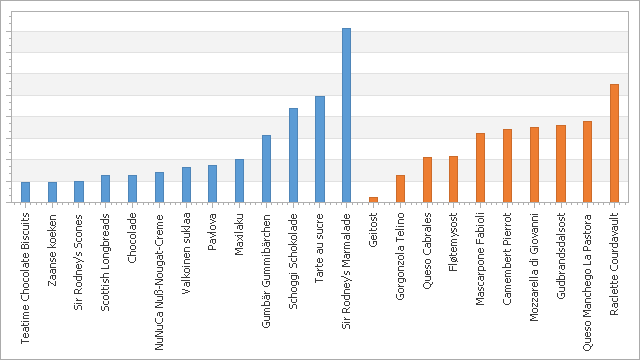• Series overlap when one series uses a secondary axis: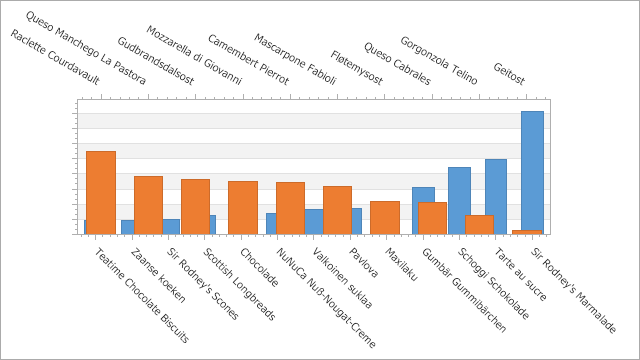• You can plot a series in a separate pane to resolve overlapping series: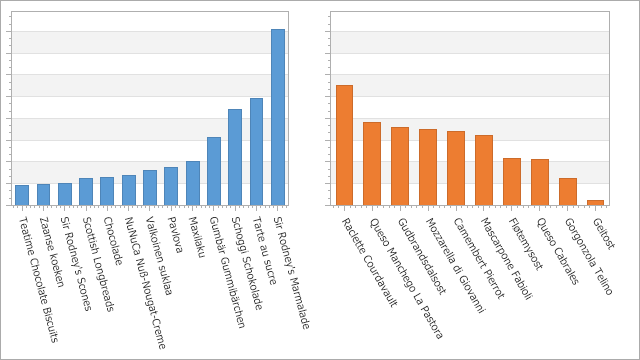• Different series' points are grouped by equal string arguments: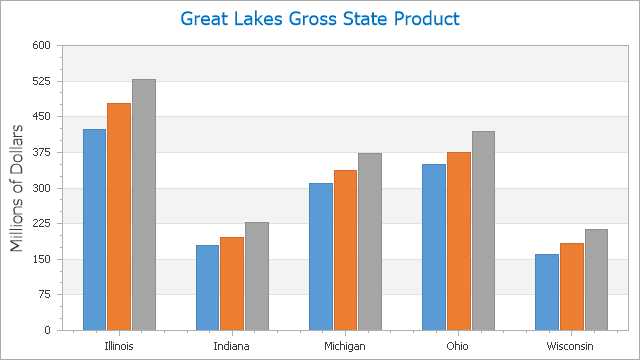### Prevent Hiding Axis Labels

The Chart Control hides axis labels if there is insufficient space. To show all string arguments for the data series when you use a qualitative scale, disable ScaleGridOptionsBase.AutoGrid, set ScaleGridOptionsBase.GridSpacing to 1, and change the Resolve Overlapping Algorithm options.

``````XYDiagram diagram = chartControl1.Diagram as XYDiagram;
diagram.AxisX.QualitativeScaleOptions.AutoGrid = false;
diagram.AxisX.QualitativeScaleOptions.GridSpacing = 1;
diagram.AxisX.Label.ResolveOverlappingOptions.AllowHide = false;
``````

### Reorder Qualitative Axis Values

You can sort qualitative values to put them in specific order. The images below show the default and custom qualitative value order.

Image Description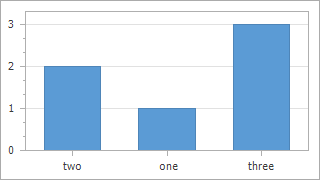The qualitative values are plotted in the same order as series points in the collection.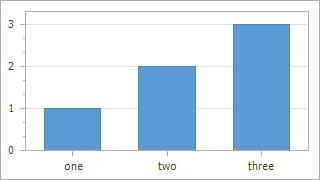The custom qualitative value order applies.

Assign an object of a class that implements the IComparer interface to the AxisBase.QualitativeScaleComparer property.

``````using System;
using System.Collections;

namespace QualitativeScaleCustomSortOrderSample {
class NumberComparer : IComparer {
public int Compare(object x, object y) {
int iX = NumberConverter.ToInt(x);
int iY = NumberConverter.ToInt(y);
return iX - iY;
}
}

class NumberConverter {
public static int ToInt(object o) {
string stringNumber = o as string;
int number = 0;
if (stringNumber == null) return -1;
if (Int32.TryParse(stringNumber, out number))
return number;
switch (stringNumber.ToLower()) {
case "one": return 1;
case "two": return 2;
case "three": return 3;
}
return number;
}
}
}
``````

## Change Intervals between Major Tick Marks

Use the ScaleGridOptionsBase.GridSpacing property to customize intervals between major tickmarks.

``````NumericScaleOptions xAxisOptions = ((XYDiagram)chartControl1.Diagram).AxisX.NumericScaleOptions;
xAxisOptions.GridSpacing = 500;
NumericScaleOptions yAxisOptions = ((XYDiagram)chartControl1.Diagram).AxisY.NumericScaleOptions;
yAxisOptions.GridSpacing = 1500;
``````

## Grid Alignment vs Grid Spacing

You can use the GridAlignment and GridSpacing properties to customize the chart's grid step.

For date-time and time-span axes, you can use the AxisLabel.TextPattern property to adjust the axis label's display format because the GridAlignment value affects the axis label's format. For example, axis labels show months if GridAlignment is set to Month.

Option #1: GridSpacing = 1; GridAlignment = Year (for the x-axis)

Option #2: GridSpacing = 12; GridAlignment = Month; AxisLabel.TextPattern = "{A:yyyy}" (for the x-axis)

Result: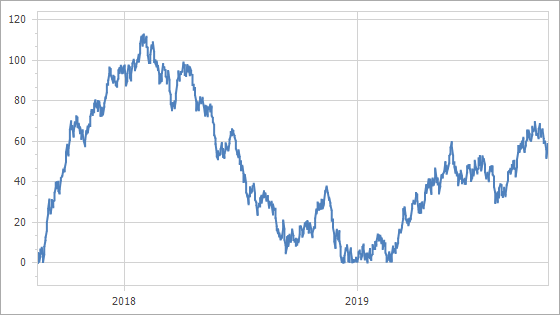For numeric axes, the GridAlignment and GridSpacing properties' values are multiplied when they are used simultaneously and the result defines the grid step.

Option #1: GridSpacing = 5; GridAlignment = NumericGridAlignment.Hundreds (for the x-axis)

Option #2: GridSpacing = 500; GridAlignment = NumericGridAlignment.Ones (for the x-axis)

Result: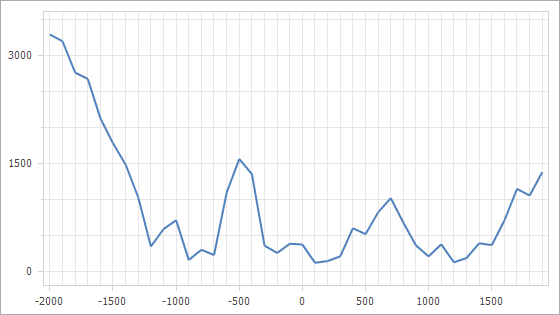## Customize Layout of Grid Lines and Axis Labels

Use the GridLayoutMode (DateTimeScaleOptions.GridLayoutMode, NumericIntervalOptions.GridLayoutMode, QualitativeScaleOptions.GridLayoutMode or TimeSpanScaleOptions.GridLayoutMode) property to rearrange grid lines, labels, and tickmarks.

``````XYDiagram diagram = chartControl1.Diagram as XYDiagram;
diagram.AxisX.DateTimeScaleOptions.GridLayoutMode = GridLayoutMode.GridShiftedLabelCentered;
diagram.AxisX.GridLines.Visible = true;
``````